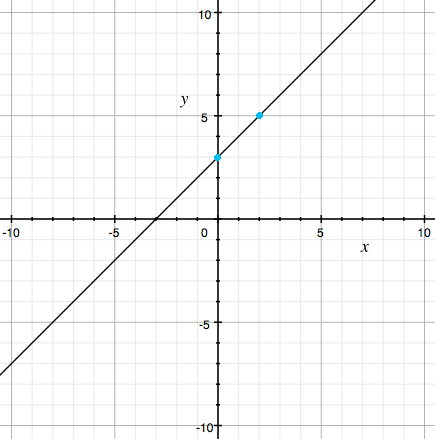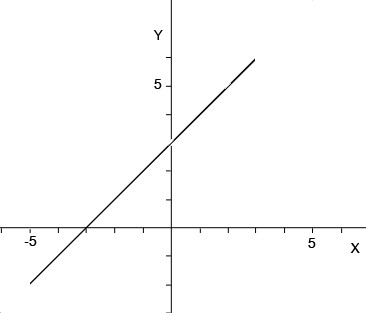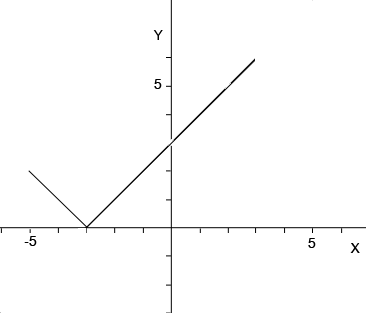SEARCH HOMEMath Central Quandaries & QueriesQuestion from Rayven, a student: Hi! I'm in eighth grade, taking ninth grade algebra 1. I'm confused as to how to graph piecewise functions. I know that you have already answered a question similar to this (I did my research first) but it didn't completely help me on my homework. I have to graph piecewise functions for the specified domains, and create a table for the absolute values. I know that two bars around a number means absolute value (two bars around -2 makes it +2) , but how do I graph and chart the absolute value for the following: f(x)= |x+3 | for -5 ≤x≤ 3 And then graph and chart: (on a separate graph): f(x)= {x if x ≤ 0         {x+1 if x < 0 thank you! ~RayvenHi Rayven,

For your first function I would begin by plotting $g(x) = x + 3.$ Hopefully you know by the form of the function the graph is a line so you need only plot two points and the draw he line that passes through them. You can choose any two points, I chose $x = 0$ which gave $f(x) = f(0) = 3$ and $x = 2$ which gave $f(x) = f(2) = 5.$ I then plotted these two points in turquoise and drew the graph of $g(v).$y = g(x) = x + 3

How does this graph change if the function is

$f(x)= |x+3 | \mbox{ for }-5 ≤x≤ 3?$

First of all $f(x)$ is defined for $-5 \leq x \leq 3$ so you need to erase the part of the graph with $x < -5$ and the part of the graph with $x > 3.$$g(x)= x+3 \mbox{ for }-5 ≤x≤ 3?$

What effect does the absolute value have on the graph? If a point $(x, g(x)),$ on the graph of $g(x)$ is on or above the X-axis then $x + 3 \geq 0$ so $f(x) = g(x)$ and the point is on the graph of $f(x).$ If a point $(x, g(x)),$ on the graph of $g(x)$ is a certain distance below the X-axis then $f(x) = -g(x)$ so the point $(x, f(x))$ is the same distance above the X-axis. Thus the piece of the graph of $g(x)$ below the X-axis is reflected in the X-axis to appear above the X-axis.$f(x)= |x+3 | \mbox{ for }-5 ≤x≤ 3?$

For your second problem I think you have one of the inequalities pointing the wrong way so I am going to change the function to

f(x)= {x if x ≤ 0
{x+1 if x > 0

would start by plotting $y = x$ and $y = x + 1$ on the same graph. You only want the piece of $y = x$ for $x \leq 0$ so erase the part of $y = x$ which has $x > 0.$ Likewise you only want the part of $y = x + 1$ with $x > 0$ so erase the part of $y = x + 1$ that has $x \leq 0.$

PennyMath Central is supported by the University of Regina and The Pacific Institute for the Mathematical Sciences.